2022 AL MVP Odds
-130
0.77 to 1Aaron Judge
35.5% implied probability

+350
3.5 to 1Shohei Ohtani
14.0% implied probability

+700
7 to 1Mike Trout
7.9% implied probability

+1000
10 to 1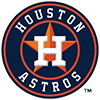Yordan Alvarez
5.7% implied probability

+1100
11 to 1Jose Ramirez
5.2% implied probability

+1300
13 to 1Rafael Devers
4.5% implied probability

+4000
40 to 1Vladimir Guerrero
1.5% implied probability

+7500
75 to 1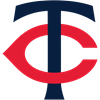Byron Buxton
0.8% implied probability

+10000
100 to 1Luis Robert
0.6% implied probability

+10000
100 to 1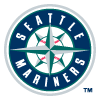Ty France
0.6% implied probability

+10000
100 to 1Tim Anderson
0.6% implied probability

+10000
100 to 1Eloy Jimenez
0.6% implied probability

+10000
100 to 1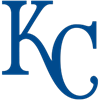Bobby Witt
0.6% implied probability

+10000
100 to 1Matt Chapman
0.6% implied probability

+10000
100 to 1Yoan Moncada
0.6% implied probability

+10000
100 to 1Alex Bregman
0.6% implied probability

+10000
100 to 1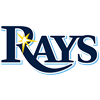Randy Arozarena
0.6% implied probability

+10000
100 to 1Kyle Tucker
0.6% implied probability

+10000
100 to 1Brandon Lowe
0.6% implied probability

+10000
100 to 1Anthony Rizzo
0.6% implied probability

+10000
100 to 1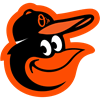Trey Mancini
0.6% implied probability

+10000
100 to 1Bo Bichette
0.6% implied probability

+10000
100 to 1Wander Franco
0.6% implied probability

+10000
100 to 1Austin Meadows
0.6% implied probability

+10000
100 to 1Michael Brantley
0.6% implied probability

+10000
100 to 1Jeimer Candelario
0.6% implied probability

+10000
100 to 1George Springer
0.6% implied probability

+10000
100 to 1Josh Donaldson
0.6% implied probability

+10000
100 to 1Giancarlo Stanton
0.6% implied probability

+10000
100 to 1DJ LeMahieu
0.6% implied probability

+10000
100 to 1Salvador Perez
0.6% implied probability

+10000
100 to 1Max Kepler
0.6% implied probability

+10000
100 to 1Jose Abreu
0.6% implied probability

+10000
100 to 1J.D. Martinez
0.6% implied probability

+10000
100 to 1Jose Altuve
0.6% implied probability

+10000
100 to 1Anthony Rendon
0.6% implied probability

+10000
100 to 1Javier Baez
0.6% implied probability

+10000
100 to 1Yasmani Grandal
0.6% implied probability

+10000
100 to 1Trevor Story
0.6% implied probability

+10000
100 to 1Xander Bogaerts
0.6% implied probability

+10000
100 to 1Carlos Correa
0.6% implied probability

+10000
100 to 1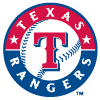Corey Seager
0.6% implied probability

+10000
100 to 1Joey Gallo
0.6% implied probability

+10000
100 to 1Mitch Haniger
0.6% implied probability

+10000
100 to 1Jesse Winker
0.6% implied probability

+10000
100 to 1Eugenio Suarez
0.6% implied probability

+10000
100 to 1Marcus Semien
0.6% implied probability

+10000
100 to 1Taylor Ward
0.6% implied probability